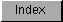```
Unlike GetSysTime(), the two values returned by ReadEClock() - tics/sec
and the EClockVal structure - have no direct relationship to actual time.
The tics/sec is the E-Clock count rate, a value which is related to the
system master clock.  The EClockVal structure is simply the upper longword
and lower longword of the E-Clock 64 bit register.

However, when two EClockVal structures are subtracted from each other and
divided by the tics/sec (which remains constant), the result does have a
relationship to actual time.  The value of this calculation is a measure
of fractions of a second that passed between the two readings.

/* E-Clock Fractions of a second fragment
*
* This fragment reads the E-Clock twice and subtracts the two ev_lo values
*         time2->ev_lo  - time1->ev_lo
* and divides the result by the E-Clock tics/secs returned by ReadEClock()
* to get the fractions of a second
*/

struct EClockVal *time1,*time2;
ULONG E_Freq;
LONG error;
struct timerequest *TimerIO;

TimerIO  = (struct timerequest *)AllocMem(sizeof(struct timerequest ),
MEMF_CLEAR | MEMF_PUBLIC);

time1 = (struct EClockVal *)AllocMem(sizeof(struct EClockVal ),
MEMF_CLEAR | MEMF_PUBLIC);

time2 = (struct EClockVal *)AllocMem(sizeof(struct EClockVal ),
MEMF_CLEAR | MEMF_PUBLIC);

if (!(error = OpenDevice(TIMERNAME,UNIT_ECLOCK,
(struct IORequest *)TimerIO,0L)) )
{
TimerBase = (struct Library *)TimerIO->tr_node.io_Device;
E_Freq =  ReadEClock((struct EClockVal *) time1); /* Get initial */

/*  place operation to be measured here */

printf("\nThe operation took: %f fractions of a second\n",
(time2->ev_lo-time1->ev_lo)/(double)E_Freq);

CloseDevice( (struct IORequest *) TimerIO );
}

The Code Takes Some Liberties.
------------------------------
The above fragment only uses the lower longword of the EClockVal
structures in calculating the fractions of a second that passed.
This was done to simplify the fragment.  Naturally, you would have to
at least check the values of the upper longwords if not use them to
get an accurate measure.

```